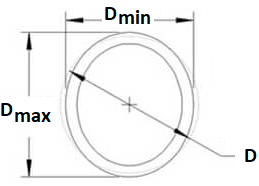Related Resources: calculators

### Ovality Roundness Tube Tube or Pipe Formulas and Calculator

Ovality (Roundness) of a Tube or Pipe Formulas and Calculator

Out of roundness, sometimes referred to as  ovality  is tube or pipe on the same cross-section of the outer diameter, roundness or ovality is difference between the maximum and minimum dimensions of outside diameter, is carefully measured the high and low points at any one section of the tube or pipe.Ovality can be calculated by the following formula:

Eq 1:

u = [ ( Dmax - Dmin) / D ] 100%

or

Eq. 2

u = [ ( Dmax - Dmin) / ( Dmax + Dmin) ] 100%

Where:

u = percent ovality of tube
Dmax = maximum outer tube diameter after bending (inch or mm)
Dmin = minimum outer tube diameter after bending (inch or mm)
D = initial outside tube diameter nominal size is often used (inch or mm)

Related: